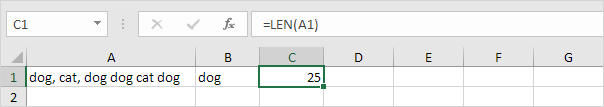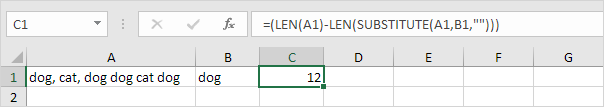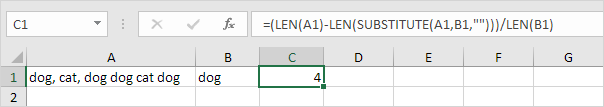# How to calculate Number of Instances in Excel

This example describes how to count the number of instances of text (or a number) in a cell.

1. Use the LEN function to get the length of the string (25 characters, including spaces).2. The SUBSTITUTE function replaces existing text with new text in a string. LEN(SUBSTITUTE(A1,B1,””)) equals 13 (the length of the string without the words dog). If we subtract this number from 25, we get the length of the dog instances (25-13=12).

Also See:   Convert text to numbers using VALUE function in Excel3. Dividing this number by the length of the word dog (3), gives us the dog instances (12/3=4).